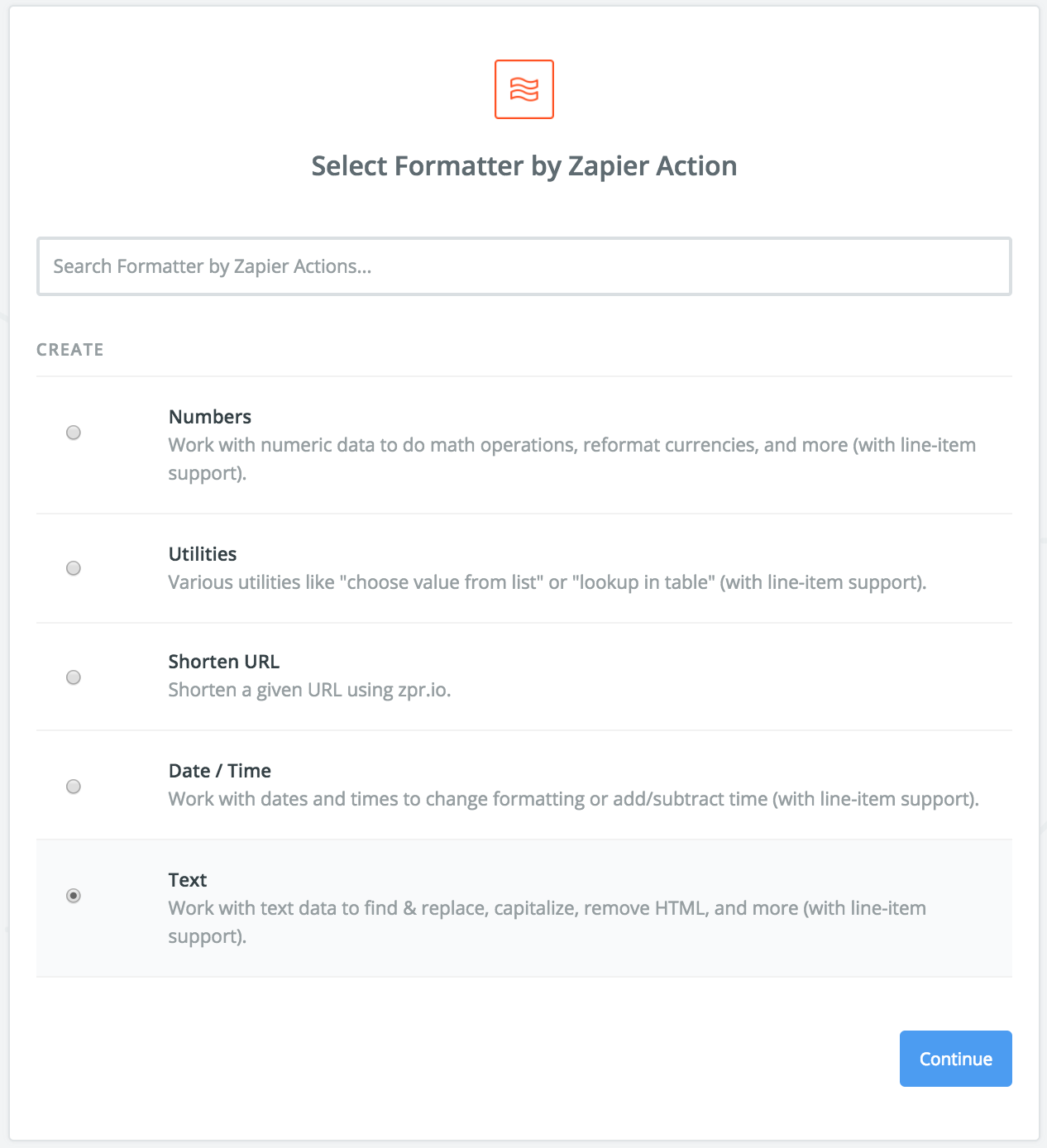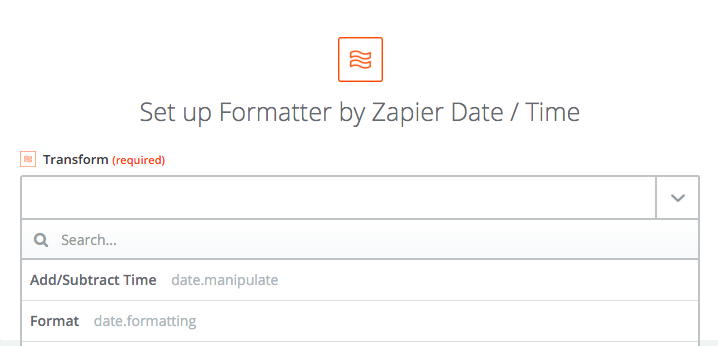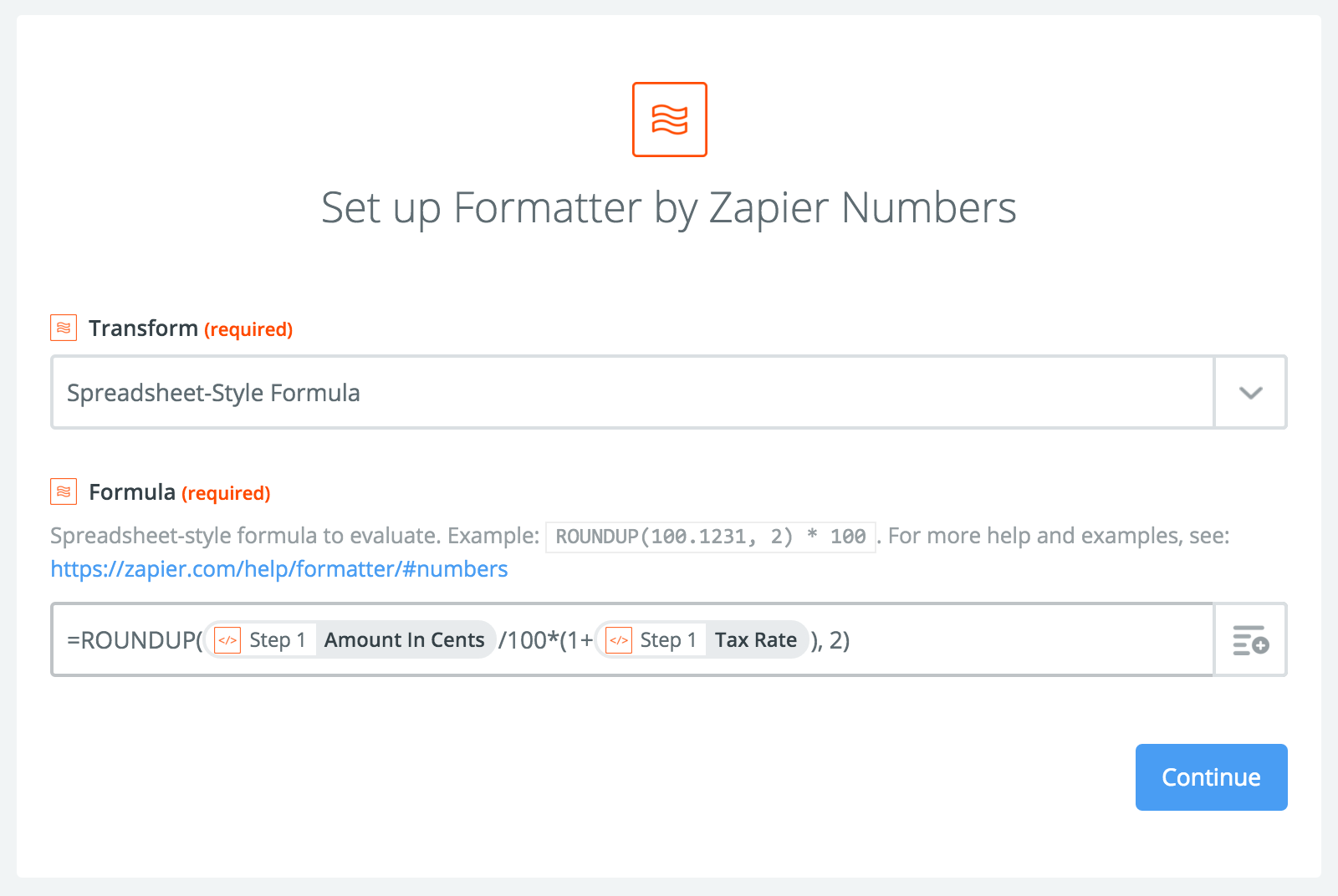# How to Get Started with Formatter by Zapier

## Connecting with Formatter by Zapier

To get started with Formatter, first add a trigger to your Zap, then add the Formatter action and select the category of data you wish to tweak:Zapier will then show you a dropdown where you select one of the available operations or transforms to perform.After selecting a transform, choose the field from your Trigger app you wish to transform, then fill in any additional options that may appear depending on the option you chose. You can test your step to verify that the action is working as expected, then add another action app to use the formatted data.

Here are the actions available for Formatter:

• Date/Time: Work with dates and times to change formatting or add/subtract time (with line-item support).
• Text: Work with text data to find replace, capitalize, remove HTML, and more (with line-item support).
• Numbers: Work with numeric data to do math operations, reformat currencies, and more (with line-item support).
• *Utilities: Various utilities like "choose value from list" or "look up in table" (with line-item support).

## Text

Formatter can clean up text and make it look perfect. Here's the tricks it can perform—and you can add multiple Formatter actions to, say, remove HTML and truncate text at the same time.

## Reformatting Text

• Capitalize: Capitalize the first character of every word.
• Lowercase: Make every character in the text lower case.
• Uppercase: Capitalize every character in a text field.
• Titlecase: Capitalize the first character of every word, except for those with fewer than 4 characters.
• Pluralize: Pluralize any English word (eg. frog turns into frogs; child turns into children).
• Replace: Replace any character, word or phrase in the text with another word or phrase.

## Extracting and Structuring Text

• Remove HTML Tags: Remove every HTML tag to leave just the plain text.
• Extract Email Address: Find and copy an email address out of a text field.
• Extract Phone Number: Find and copy any phone number (including area and country codes) out of a text field.
• Extract URL: Find and copy a URL out of a text field.
• Extract Number: Find and copy any number out of a text field.
• Extract Pattern: Find the first match for a regular expression in a text field. Returns all matched groups with start and end position.

## Encoding and Decoding Text

• URL Encode: Encodes text for use in URLs.
• URL Decode: Decodes text from URLs.
• Convert Markdown to HTML: Convert Markdown formatted text into HTML. Supports full GitHub-style Markdown syntax.

## Editing Text

• Split Text: Split your content at a specified separator (e.g., a comma). You can use `[:newline:]` to split text separated by line breaks.
• Trim Whitespace: Remove extra spaces at the end of the text.
• Length: Count the number of characters in a field.
• Word Count: Count the number of words in a text field.

## Other Text Transforms

• Convert to ASCII: Replaces all non-ASCII or special characters in a text field with normal characters.
• Default Value: Return a default value if the text is empty.
• Find: Find a set value in a field and optionally set a default value if nothing is found.
• Superhero Name: Convert a name into the name of a Superhero.
• Truncate: Limit your text to a specific character length, and delete anything over that.

## Special Characters

Sometimes you want to find/replace or split on special characters. For these cases, you can use a special character syntax:

• `[:space:]` - matches space (`\s`) characters
• `[:tab:]` - matches tab (`\t`) characters
• `[:newline:]` - matches newline (`\n`) characters
• `[:return:]` - matches carriage-return (`\r`) characters

## Numbers

Numbers may not need any conversion to work correctly in your app, but they might need formatting to help them look nicer—and some automated math might make your job easier. Formatter includes these number tools:

• Format Currency: Format a number into a specific currency, locale, and format style.
• Format Number: Choose the symbol used for grouping and decimal place in a number. Does not perform any rounding or padding of the number.
• Format Phone Number: Format a phone number into a specific style.
• Perform Math Operation: Add, subtract, multiply, or divide values, or make a number negative (Does not support Line-item fields)
• Spreadsheet-Style Formula: Evaluate a formula similar to formulas found in popular spreadsheet applications.

With math operations, choose the fields you wish to add, subtract, multiply, or divide, and Formatter will perform the operation without any extra input. For more advanced math, try using Zapier's Code integration.Spreadsheet-Style Formula allows you to evaluate a formula with the same syntax found in popular spreadsheet applications, like Excel, Numbers, and Google Sheets. Your formulas have the ability to perform arithmetic operations, make comparisons, and call a set of functions.

Please note, if you are trying to evaluate data that is text, and not numbers, you will need to wrap any and all text strings in "double quotes" otherwise the formatter will return with an error.

## Formula Examples

• `(1 + 2) / 4` returns `0.75`
• `POW(2, 4)` returns `16`
• `IF(ISEVEN(2), 100, 200)` returns `100`
• `AND(ISODD(1), ISEVEN(2))` returns `TRUE`
• `ROUNDUP(1234.567 * 106%, 2)` returns `1308.65`
• `IF(1 2, IF(3 = 3, "you did it!", 20), 30)` returns `you did it!`
• `100 * (VALUE("123" "." "01") + 7)` returns `13001`

## Formula Functions

ABS(number)
Returns the absolute value of a number
ACOS(number)
Returns the inverse cosine of a number
ACOSH(number)
Returns the inverse hyperbolic cosine of a number
AND(condition, [condition, ...])
Returns TRUE if all of the conditions evaluate to TRUE, otherwise FALSE
ASIN(number)
Returns the inverse sine of a number
ASINH(number)
Returns the inverse hyperbolic sine of a number
ATAN(number)
Returns the inverse tangent of a number
ATAN2(x, y)
Returns the inverse tangent of a pair of x and y coordinates
ATANH(number)
Returns the inverse hyperbolic tangent of a number
AVERAGE(number, [number, ...])
Returns the average of a list of numbers
CEILING(number, [factor])
Returns a number rounded up to the nearest multiple of factor (e.g., `CEILING(3.14159, 0.1) = 3.2` and `CEILING(3.14159, 2) = 4`)
COS(number)
Returns the cosine of a number
COSH(number)
Returns the hyperbolic cosine of a number
Returns radians converted to a value in degrees
EVEN(number)
Rounds a number up to the next even number
EXP(number, power)
Returns the constant e raised to a power
FACT(number)
Returns the factorial of a number
FACTDOUBLE(number)
Returns the double factorial of a number
FALSE()
Returns the logical value FALSE
FLOOR(number, [factor])
Returns a number rounded down to the nearest multiple of factor (e.g., `FLOOR(3.14159, 0.1) = 3.1` and `FLOOR(3.14159, 2) = 2`)
GCD(number, number, [number, ...])
Returns the greatest common divisor of two or more numbers
GEOMEAN(number, [number, ...])
Returns the geometric mean of a list of numbers
IF(condition, true_value, [false_value])
Returns the true_value if the condition is TRUE or false_value if the condition is FALSE
INT(number)
Returns a number rounded down to the previous integer
ISBLANK(value)
Returns TRUE if a value is blank (empty); Otherwise, returns FALSE
ISEVEN(value)
Returns TRUE if a value is an even number; Otherwise, returns FALSE.
ISLOGICAL(value)
Returns TRUE if a value is a logical value; Otherwise, returns FALSE
ISNONTEXT(value)
Returns TRUE if a value is NOT text; Otherwise, returns FALSE
ISNUMBER(value)
Returns TRUE if a value is a number; Otherwise, returns FALSE
ISODD(value)
Returns TRUE if a value is an odd number; Otherwise, returns FALSE
ISTEXT(value)
Returns TRUE if a value is text; Otherwise, returns FALSE
LCM(number, number, [number, ...])
Returns the least common multiple of two or more numbers
LN(number)
Returns the natural logarithm of a number
LOG(number, base)
Returns the logarithm of a number, to a specified base
LOG10(number)
Returns the base 10 logarithm of a number
MAX(number, [number, ...])
Returns the largest value from a list of numbers
MEDIAN(number, [number, ...])
Returns the median (the middle value) of a list of numbers
MIN(number, [number, ...])
Returns the smallest value from a list of numbers
MOD(dividend, divisor)
Returns the remainder from a division between two numbers
MODE(number, [number, ...])
Returns the mode (the most frequently occurring value) of a list of numbers
NOT(condition)
Returns a logical value that is the opposite of a condition (ie. returns FALSE if condition is TRUE and returns TRUE if condition is FALSE)
ODD(number)
Returns a number rounded up to the next odd number
OR(condition, [condition, ...])
Returns TRUE if any of the conditions evaluate to TRUE, otherwise FALSE
PI()
Returns the constant value of Pi
POW(number, power)
Returns the result of a number raised to a power
POWER(number, power)
Returns the result of a number raised to a power
PRODUCT(number, [number, ...])
Returns the product of a list of numbers
QUOTIENT(dividend, divisor)
Returns the integer portion of a division between two numbers
Returns degrees converted to a value in radians
RAND()
Returns a random number between 0 and 1
RANDBETWEEN(min, max)
Returns a random number between two integers
ROUND(number, [places])
Returns a number rounded up or down (following standard rules) to a certain number of decimal places
ROUNDDOWN(number, [places])
Returns a number rounded up to a certain number of decimal places
ROUNDUP(number, [places])
Returns a number rounded up to a certain number of decimal places
SIGN(number)
Returns the sign (+, -, 0) of a number
SIN(number)
Returns the sine of a number
SINH(number)
Returns the hyperbolic sine of a number
SQRT(number)
Returns the positive square root of a number
SQRTPI(number)
Returns the square root of a number multiplied by the constant Pi
SUM(number, [number, ...])
Returns the sum of a list of numbers
TAN(number)
Returns the tangent of a number
TANH(number)
Returns the hyperbolic tangent of a number
TRUE()
Returns the logical value TRUE
TRUNC(number, [places])
Returns a number truncated to a certain number of significant digits by omitting less significant digits
VALUE(text)
Returns the text converted to a numeric value

## Formula Arithmetic

Negation Operator
`-A` converts A to a negative number
Percent Operator
`A%` converts A to a percentage value by dividing it by 100
Exponentiation Operator
`A ^ B` raises A number to the power of B
Multiplication Operator
`A * B` multiplies A by B
Division Operator
`A / B` divides A by B
`A + B` adds B to A
Subtraction Operator
`A - B` subtracts B from A

## Formula Comparisons

Equal Condition
`A = B` is TRUE when A is equal to B
Not Equal Condition
`A <> B` is TRUE when A is not equal to B
Greater Than Condition
`A > B` is TRUE when A is greater than B
Less Than Condition
`A < B` is TRUE when A is less than B
Greater Than or Equal Condition
`A >= B` is TRUE when A is greater than or equal to B
Less Than or Equal Condition
`A <= B` is TRUE when A is less than or equal to B

## Date Time

Dates can be tricky when moving between apps since each app has its own way of listing dates and times. These actions can help you get them in the format you need—and can let you add or subtract time to account for time zones and more.

### Format - Change the format and time zone of a timestamp

To switch a timestamp to a different format or time zone from the one sent on, choose the Format utility from the dropdown. Then, insert the timestamp from the trigger app, and select the format the date is currently in, and the new format you want to use. If the format you want isn't available, select the Custom tab and create your own format by typing it in. Choose the format by referencing the table below:

### Date/Time Custom Value Table

Token Output
Year YYYY 2000, 2001, 2002 ... 2012, 2013
YY 00, 01, 02 ... 12, 13
Month MMMM January, February, March ...
MMM Jan, Feb, Mar ...
MM 01, 02, 03 ... 11, 12
M 1, 2, 3 ... 11, 12
Day of Year DDDD 001, 002, 003 ... 364, 365
DDD 1, 2, 3 ... 364, 365
Day of Month DD 01, 02, 03 ... 30, 31
D 1, 2, 3 ... 30, 31
Day of Week dddd Monday, Tuesday, Wednesday ...
ddd Mon, Tue, Wed ...
d 1, 2, 3 ... 6, 7
Hour HH 00, 01, 02 ... 23, 24
H 0, 1, 2 ... 23, 24
hh 01, 02, 03 ... 11, 12
h 1, 2, 3 ... 11, 12
AM / PM A AM, PM
a am, pm
Minute mm 00, 01, 02 ... 58, 59
m 0, 1, 2 ... 58, 59
Second ss 00, 01, 02 ... 58, 59
s 0, 1, 2 ... 58, 59
Sub-second SSS 000, 001, 002 ... 998, 999
SS 00, 01, 02 ... 98, 99
S 0, 1, 2 ... 8, 9
Timezone ZZ -07:00, -06:00 ... +06:00, +07:00
Z -0700, -0600 ... +0600, +0700
Timestamp X 1381685817

### Add/Subtract Time - Manipulate a date and/or time by adding/subtracting time

To adjust a date/time by a specific amount, use + or -. Units can be "years", "months", "weeks", "days", "hours", "minutes", or "seconds". Here are some examples:

• +1 hour
• -3 days
• +5 years +2 months -3 minutes
• +8hNote

This syntax is different from the syntax you would use to [modify a date/time in a date/time field](https://zapier.com/help/modifying-dates-and-times/#adjusting-dates-and-times). The examples above apply only to the Add/Subtract Time utility in Formatter.

## Other Utilities

Formatter also includes other tools:

Need to reformat text, numbers, or dates into another format? Code by Zapier lets you tweak any fields from your apps with Javascript or Python code.

0 out of 0 found this helpful
•### Contact Support

Get help from Zapier Support. Contact Support
•### Visit Community

Find answers and inspiration. Visit Community
•### Hire an Expert

Leverage professionals across the globe ready to help. Hire an Expert
•### Zapier Learn

Take courses designed to help you become a better Zapier user. Zapier Learn